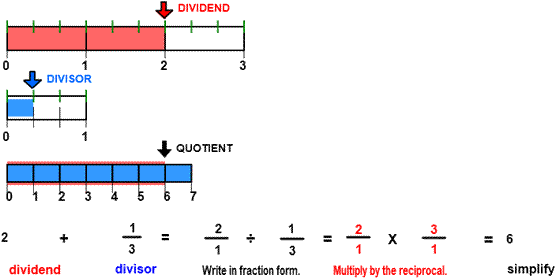DIVIDE
CORRECT:
ATTEMPTS:
SCORE:
PERCENT
WHOLE
NUMERATOR
DENOMINATOR

# DIVIDE FRACTIONS INSTRUCTIONS

Follow the directions in the dialog box after pressing the <START> button. The <EXPLAIN> button may then be pressed after you enter the divisor to see how to do the example.

The following image was made from Divide with Lines Designer:The parts of a division example are the dividend, the divisor, and the quotient.

When the program starts, you will be asked to identify the dividend. The dividend is the horizontal distance of the red arrow.

Once the dividend is correctly identified, a rectangle representing the divisor will appear. The divisor is the horizontal distance of the blue arrow. The program will not continue unless the dividend or the divisor are correctly identified.

You will then be asked to find the quotient. The quotient is the number of blue divisor lengths that will fit into the red dividend length.

You can see from the image that 6 divisor lengths will fit into the dividend length. The quotient, then, is 6. Many of the quotients in this program can by arrived at by inspecting the images. How many divisor lengths will fit into the dividend length?

All the examples in this program will have a whole number quotient from 1 to 12.

To calculate the quotient,  first write the dividend and the divisor in fraction form as shown in the example above. Then multiply the dividend (21) by the inverse of the divisor(12). You can divide by 13 by multiplying by 31. 31 is the inverse (or reciprocal) of 13.

See MULTIPLY FRACTIONS  for instructions on how to multiply fractions.

See MULTIPLICATIVE INVERSE for more information on how to find the inverse.

For more instruction on dividing fractions go to How To Divide Fractions

After you enter the quotient you may press the <REPORT> button. The report will ask for your name but you may submit a code for your name. This report will give the same results as on the dialog box. The report may be printed or e-mailed.